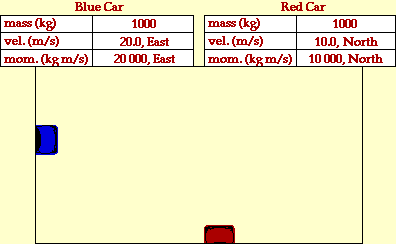## Two Cars in 2-Dimensional Collision

### Inelastic Collision

Collisions between objects are governed by laws of momentum and energy. When a collision occurs in an isolated system, the total momentum of the system of objects is conserved. Provided that there are no net external forces acting upon the objects, the momentum of all objects before the collision equals the momentum of all objects after the collision. If there are only two objects involved in the collision, then the momentum change of the individual objects are equal in magnitude and opposite in direction.

Certain collisions are referred to as elastic collisions. Elastic collisions are collisions in which both momentum and kinetic energy are conserved. The total system kinetic energy before the collision equals the total system kinetic energy after the collision. If total kinetic energy is not conserved, then the collision is referred to as an inelastic collision.

The animation below portrays the inelastic collision between two 1000-kg cars. The before- and after-collision velocities and momentum are shown in the data tables.In the collision between the two cars, total system momentum is conserved. Yet this might not be apparent without an understanding of the vector nature of momentum. Momentum, like all vector quantities, has both a magnitude (size) and a direction. When considering the total momentum of the system before the collision, the individual momentum of the two cars must be added as vectors. That is, 20 000 kg*m/s, East must be added to 10 000 kg*m/s, North. The sum of these two vectors is not 30 000 kg*m/s; this would only be the case if the two momentum vectors had the same direction. Instead, the sum of 20 000 kg*m/s, East and 10 000 kg*m/s, North is 22 361 kg*m/s at an angle of 26.6 North of East. Since the two momentum vectors are at right angles, their sum can be found using the Pythagorean theorem; the direction can be found using SOH CAH TOA (specifically, the tangent function). The value 22 361 kg*m/s is the total momentum of the system before the collision; and since momentum is conserved, it is also the total momentum of the system after the collision. Since the cars have equal mass, the total system momentum is shared equally by each individual car. In order to determine the momentum of either individual car, this total system momentum must be divided by two (approx. 11 200 kg*m/s). Once the momentum of the individual cars are known, the after-collision velocity is determined by simply dividing momentum by mass (v=p/m).

An analysis of the kinetic energy of the two objects reveals that the total system kinetic energy before the collision is 250 000 Joules (200 000 J for the eastbound car plus 50 000 J for the northbound car). After the collision, the total system kinetic energy is 125 000 Joules (62 500 J for each car). The total kinetic energy before the collision is not equal to the total kinetic energy after the collision. A large portion of the kinetic energy is converted to other forms of energy such as sound energy and thermal energy. A collision in which total system kinetic energy is not conserved is known as an inelastic collision.

For more information on physical descriptions of motion, visit The Physics Classroom Tutorial. Detailed information is available there on the following topics:

Momentum

Momentum Conservation Principle

Isolated Systems

Momentum Conservation in Collisions

Kinetic Energy

Use of Pythagorean Theorem and SOH CAH TOA to Find the Sum (Resultant) of Two Vectors